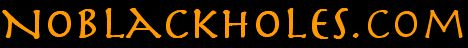Conceptual Papers
(no equations):

Technical Papers:

Other Articles & Papers:

## A brief history of black holes

Isaac Newton's seminal work on gravity, Principia (1687), postulates a law of gravity where gravitational potential energy outside a mass is inversely proportional to radial distance from the center of the mass. According to this law of gravity, the escape velocity on the surface of a mass increases as the mass is compacted. In 1783 the Reverend John Michell, a British natural philosopher, pointed out that if a mass could be compacted within a critical radius where the escape velocity on the surface of the mass equals the speed of light, light would not escape from the surface. This would create an an invisible mass, now called a black hole.

In 1916, Karl Schwarzschild solved Einstein's field equations to produce an equation, called the Schwarzschild metric, that describes the effect gravity has on space and time outside a compacted mass. The Schwarzschild metric has a singularity at the critical radius where the Newtonian escape velocity equals the speed of light.  In mathematics, a singularity is a point at which an equation either blows up to infinity or degenerates to zero.  The physical implication of the singularity in the Schwarzschild metric is that it takes an infinite amount of time, as measured from any starting location outside the critical radius, for anything (light or matter) to reach the critical radius. This effectively makes the inner volume defined by the critical radius  impenetrable,  delineates a maximum density for a mass, and thereby prevents formation of a black hole.

Karl Schwarzschild explained why the maximum density delineated by the critical radius is a real physical limitation.  Albert Einstein defended this position in a paper on this topic in 1939. During Einstein's lifetime very few challenged this view.

After Einstein was no longer around to provide a defense, Schwarzschild’s view was challenged based on the assertion that there is no barrier to a freefalling particle travelling past the critical radius, thereby allowing matter to compact into a black hole.  However, the calculations relied upon to get the particle across the critical radius violate ordinary rules of mathematics, logic and, most notably, the very conservation laws that serve as the foundation of Einstein's theory of general relativity.

Louise de Broglie, a French physicist and Nobel laureate, quoted Einstein as observing "that all physical theories, their mathematical expressions apart, ought to lend themselves to so simple a description 'that even a child could understand.'"

This website, in the spirit of Einstein's belief in the elegance of the laws governing creation, explains why black holes are incompatible with Einstein's theory of general relativity and point out some of the shenanigans that have been used to link the notion of a black hole to Einstein's theories.

Read more about: The time barrier that prevents formation of black holes.